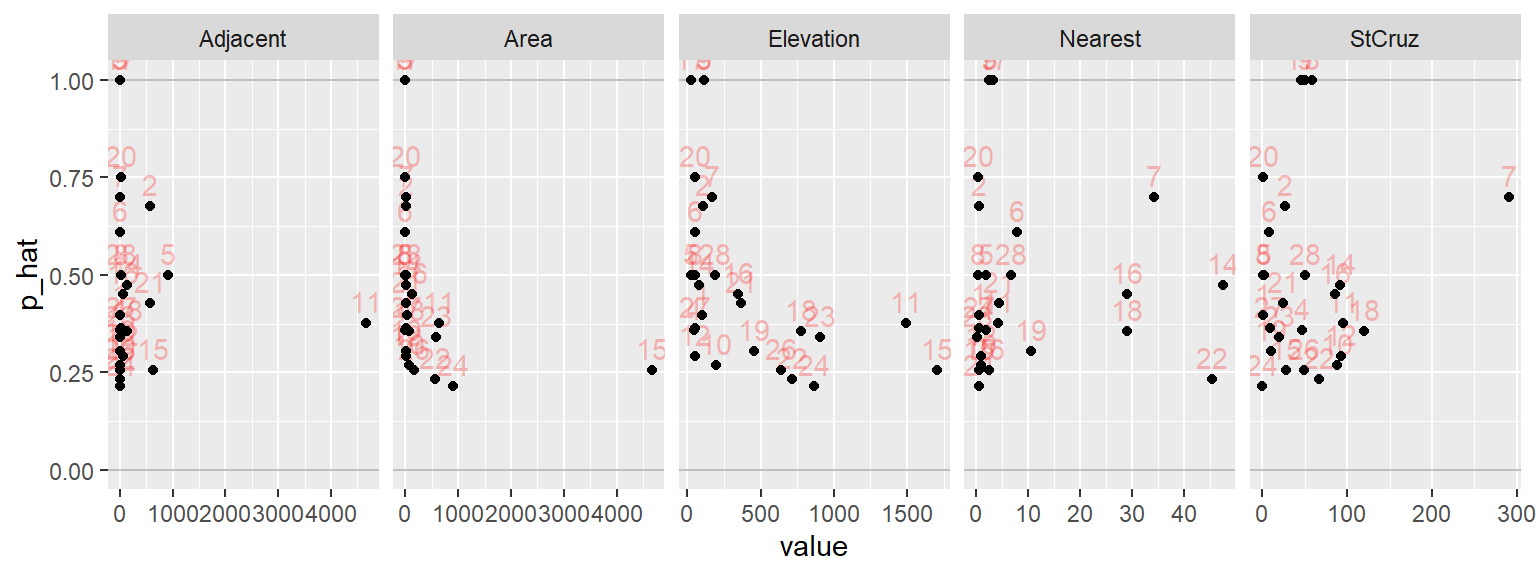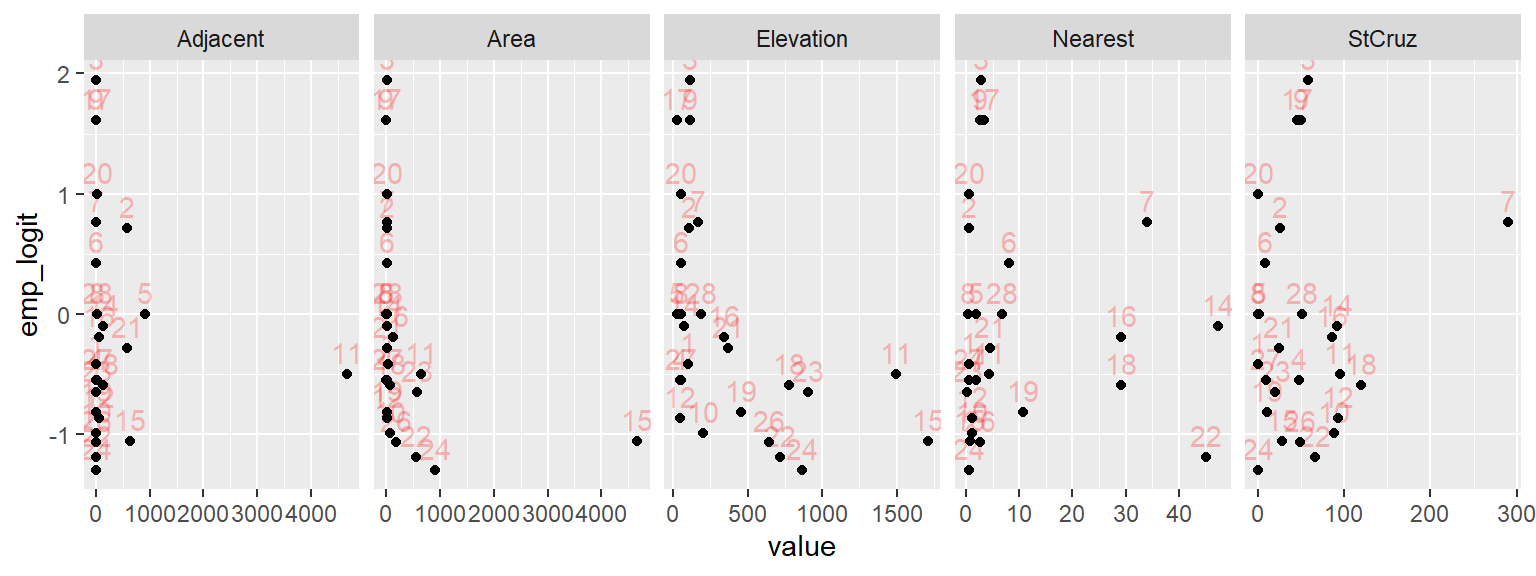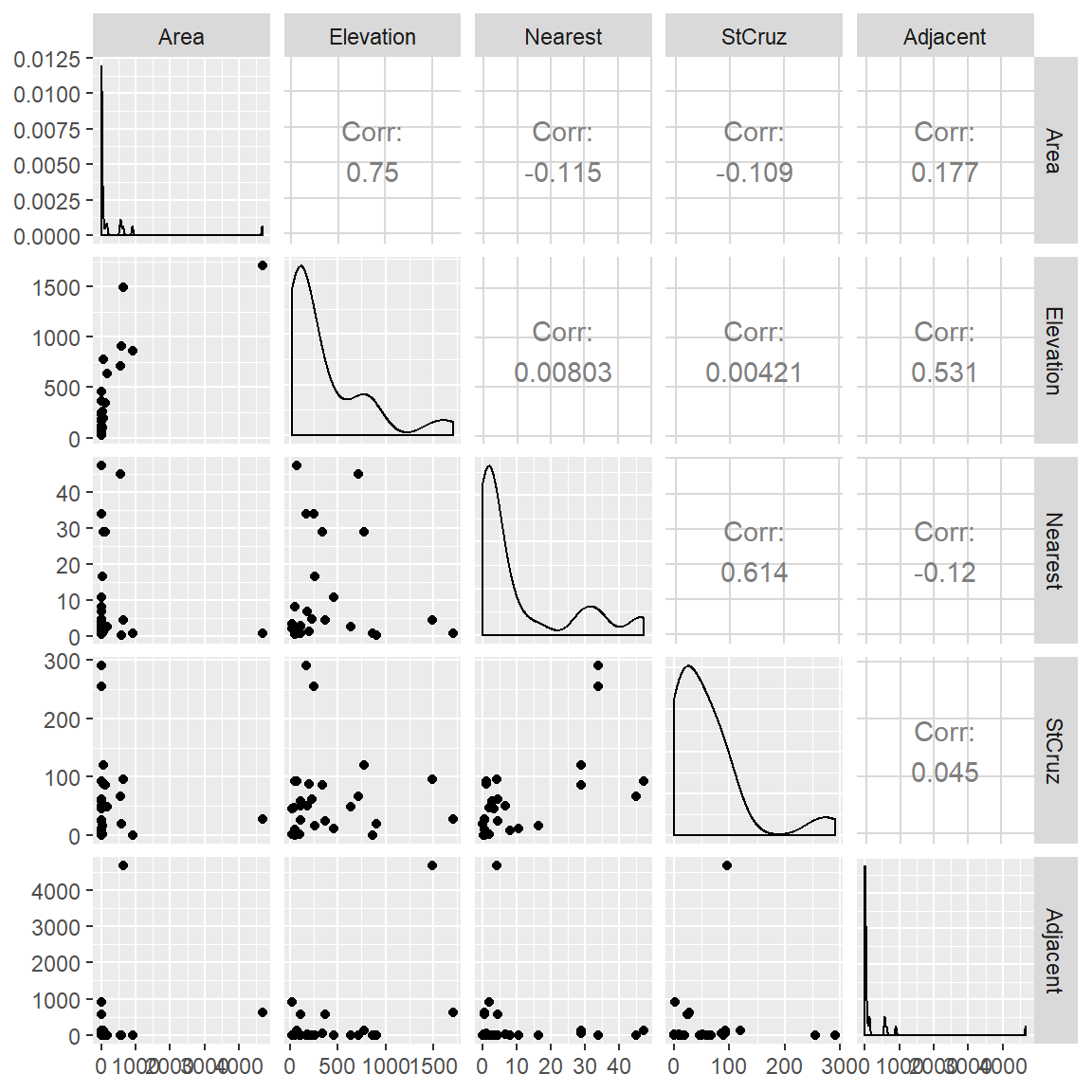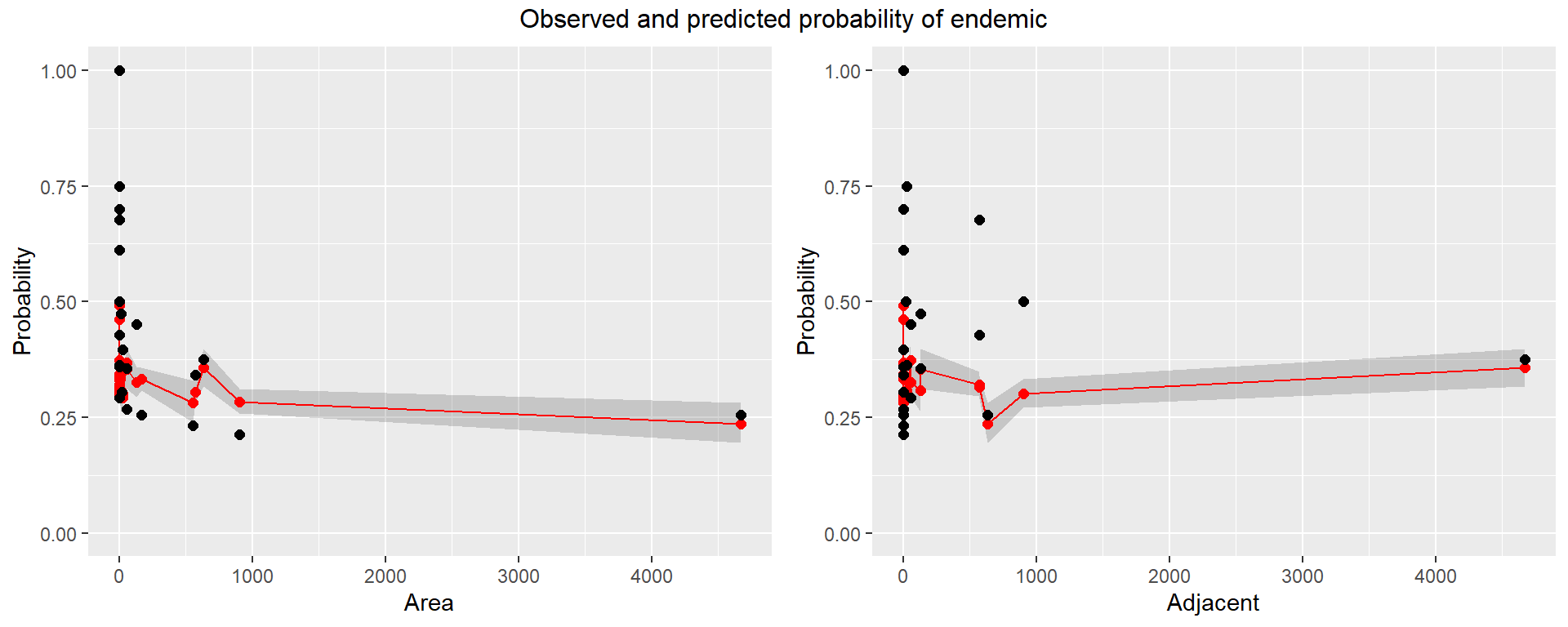# 1Galapagos Island Species Data

The Galapagos Islands about 600 miles off the coast of Ecuador provide an excellent laboratory for studying the factors that influence the development and survival of different life species. They were the site of much of Charles Darwin’s original research leading later to publication of his “Origin of Species”. Descending from a few stranded ancestors and cut off from the rest of the world, the Galapagos animals offer much more obvious proofs of the fact of evolution than can be seen in the more intricate complexities of life in most environments. Darwin wrote:

The natural history of these islands is eminently curious, and well deserves attention. Most of the organic productions are aboriginal creations, found nowhere else; there is even a difference between the inhabitants of the different islands; yet all show a marked relationship with those of America, though separated from that continent by an open space of ocean, between 500 and 600 miles in width. The archipelago is a little world in itself, or rather a satellite attached to America, whence it has derived a few stray colonists and has received the general character of its indigenous productions. Considering the small size of the islands, we feel the more astonished at the number of their aboriginal beings, and at their confined range. Seeing every height crowned with its crater, and the boundaries of most of the lava-streams still distinct, we are led to believe that within a period geologically recent the unbroken ocean was here spread out. Hence, both in space and time, we seem to be brought somewhere near to that great fact—that mystery of mysteries—the first appearance of new beings on earth.

And from elsewhere in Darwin’s diary: I never dreamed that islands 50 or 60 miles apart, and most of them in sight of each other, formed of precisely the same rocks, placed under a quite similar climate, rising to a nearly equal height, would have been differently tenanted… It is the circumstance that several of the islands possess their own species of the tortoise, mocking-thrush, finches and numerous plants, these species having the same general habits, occupying analogous situations, and obviously filling the same place in the natural economy of the archipelago, that strikes me with wonder.

M.P. Johnson and P.H. Raven, “Species number and endemism: The Galapagos Archipelago revisited”, Science, 179, 893-895 (1973), have presented data giving the number of plant species and related variables for 29 different islands. Counts are given for both the total number of species and the number of species that occur only in the Galapagos (the endemics). Elevations for Baltra and Seymour obtained from web searches. Elevations for four other small islands obtained from large-scale maps.

Variable    Description
Island      Name of Island
Plants      Number of plant species
PlantEnd    Number of endemic plant species
Finches     Number of finch species
FinchEnd    Number of endemic finch species
FinchGenera Number of finch genera
Area        Area (km^2)
Elevation   Maximum elevation (m)
Nearest     Distance from to nearest island (km)
StCruz      Distance to Santa Cruz Island (km)
Adjacent    Area of adjacent island (km^2)

Goal: To build a model to predict the proportion of endemic plants on an island based on the island characteristics.

library(tidyverse)

#   save in the same folder as this Rmd file.

dat_gal <-
, skip = 27             # I was expecting to skip 28, not sure why it wants 27
) %>%
mutate(
id = 1:n()
)

Compute the observed proportion and empirical logits of endemic plants on each island.

# observed proportions
dat_gal <-
dat_gal %>%
mutate(
p_hat     = PlantEnd / Plants
# emperical logits
, emp_logit = log((p_hat + 0.5/Plants) / (1 - p_hat + 0.5/Plants))
)

Artificially remove the responses for three islands to predict later.

# list of islands to predict
island_pred_list <-
c(
"Gardner2"
, "Santa.Fe"
, "Wolf"
)

## capture the observed probabilities
dat_gal_pred_true <-
dat_gal %>%
filter(
Island %in% island_pred_list
)

# Set these islands with missing response variables
#  (there must be a better way to NA selected rows, but I didn't find it)
dat_gal <-
dat_gal %>%
mutate(
Plants    = ifelse(Island %in% island_pred_list, NA, Plants   )
, PlantEnd  = ifelse(Island %in% island_pred_list, NA, PlantEnd )
, p_hat     = ifelse(Island %in% island_pred_list, NA, p_hat    )
, emp_logit = ifelse(Island %in% island_pred_list, NA, emp_logit)
)

Data modifications

## RETURN HERE TO SUBSET AND TRANSFORM THE DATA

dat_gal <-
dat_gal %>%
mutate(
) %>%
filter(
TRUE  # !(id %in% c( ... ))
)

### SOLUTION

## 1.1(2 p) Interpret plot of observed proportions against predictors

# Create plots for proportion endemic for each variable
dat_gal_long <-
dat_gal %>%
select(
Island, id, p_hat, emp_logit, Area, Elevation, Nearest, StCruz, Adjacent
) %>%
pivot_longer(
cols = c(Area, Elevation, Nearest, StCruz, Adjacent)
, names_to  = "variable"
, values_to = "value"
)

# Plot the data using ggplot
library(ggplot2)
p <- ggplot(dat_gal_long, aes(x = value, y = p_hat, label = id))
p <- p + geom_hline(yintercept = c(0,1), alpha = 0.25)
p <- p + geom_text(hjust = 0.5, vjust = -0.5, alpha = 0.25, colour = 2)
p <- p + geom_point()
p <- p + scale_y_continuous(limits = c(0, 1))
p <- p + facet_wrap( ~ variable, scales = "free_x", nrow = 1)
print(p)# Plot the data using ggplot
library(ggplot2)
p <- ggplot(dat_gal_long, aes(x = value, y = emp_logit, label = id))
p <- p + geom_text(hjust = 0.5, vjust = -0.5, alpha = 0.25, colour = 2)
p <- p + geom_point()
p <- p + facet_wrap( ~ variable, scales = "free_x", nrow = 1)
print(p)Comment on how the proportion of endemic plants depends on each variable (in terms of increase/decrease).

Also, interpret the plot regarding whether the empirical logits appear linear (or any trends). Note that the marginal empirical logit plots do not have to be linear, but the model in 6-dimensional space should be roughly “linear”.

Indicate whether any observations are gross outliers and should be dropped, and whether variables are obvious candidates for transformation. Then, drop outliers, transform and update your comments, and repeat until satisfied.

### 1.1.1 Solution

Proportion plots:

Empirical logit plots:

## 1.2(0 p) Predictor scatterplot matrix

For further information, the relationship between predictors is plotted.

# relationships between predictors

library(ggplot2)
library(GGally)
p <- ggpairs(dat_gal %>% select(Area, Elevation, Nearest, StCruz, Adjacent))
print(p)## 1.3(1 p) Fit a logistic regression model, interpret deviance lack-of-fit

Fit a logistic model relating the probability of endemic plants to the predictors. Decide which predictors to use.

  ### SOLUTION
# Don't include both Area and Elevation, since highly correlated

glm_g_aensj <-
glm(
cbind(PlantEnd, Plants - PlantEnd) ~ Area + Nearest + StCruz + Adjacent
, family = binomial
, data = dat_gal
)

# Test residual deviance for lack-of-fit (if > 0.10, little-to-no lack-of-fit)
dev_p_val_full <- 1 - pchisq(glm_g_aensj$deviance, glm_g_aensj$df.residual)
dev_p_val_full
 3.217315e-12
## Stepwise selection
# option: trace = 0 doesn't show each step of the automated selection
glm_g_aensj_red_AIC <-
step(
glm_g_aensj
, direction = "both"
, trace = 0
)

# the anova object provides a summary of the selection steps in order
glm_g_aensj_red_AIC$anova  Step Df Deviance Resid. Df Resid. Dev AIC 1 NA NA 21 99.73305 208.5214 2 - Adjacent 1 1.72817 22 101.46122 208.2495 coef(glm_g_aensj_red_AIC)  (Intercept) Area Nearest StCruz -8.357063e-01 -9.264229e-05 -6.121220e-03 3.490689e-03  # Test residual deviance for lack-of-fit (if > 0.10, little-to-no lack-of-fit) dev_p_val_red <- 1 - pchisq(glm_g_aensj_red_AIC$deviance, glm_g_aensj_red_AIC$df.residual) dev_p_val_red  3.579248e-12 Look at the residual deviance lack-of-fit statistic for the full model. Is there evidence of any gross deficiencies with the model? How about for the reduced model? (Regardless of lack of fit result, continue with the assignment. This is a realistic example and not everything may work out nicely.) ### 1.3.1 Solution [answer] Full: Reduced: ## 1.4(2 p) Interpret logistic regression coefficients Which variables appear to be a useful predictor of the probability of endemic plants? Interpret the hypothesis test(s). summary(glm_g_aensj_red_AIC)  Call: glm(formula = cbind(PlantEnd, Plants - PlantEnd) ~ Area + Nearest + StCruz, family = binomial, data = dat_gal) Deviance Residuals: Min 1Q Median 3Q Max -3.382 0.223 1.182 2.063 4.056 Coefficients: Estimate Std. Error z value Pr(>|z|) (Intercept) -8.357e-01 7.642e-02 -10.935 < 2e-16 *** Area -9.264e-05 3.173e-05 -2.920 0.00350 ** Nearest -6.121e-03 3.341e-03 -1.832 0.06694 . StCruz 3.491e-03 1.333e-03 2.619 0.00881 ** --- Signif. codes: 0 '***' 0.001 '**' 0.01 '*' 0.05 '.' 0.1 ' ' 1 (Dispersion parameter for binomial family taken to be 1) Null deviance: 119.39 on 25 degrees of freedom Residual deviance: 101.46 on 22 degrees of freedom (3 observations deleted due to missingness) AIC: 208.25 Number of Fisher Scoring iterations: 3 ### 1.4.1 Solution [answer] XXX is significant and it’s negative coefficient of -9.2610^{-5} suggests that as XXX increases, the proportion of endemic plants decreases. etc. ## 1.5(1 p) Write model equation Provide an equation relating the fitted probability of endemic plants to the selected predictor variables on the probability scale. ### 1.5.1 Solution [answer] The logistic equation is $\tilde{p} = \frac{ \exp( XXX )} { 1 + \exp( XXX ) } .$ ## 1.6(0 p) Plot the fitted probabilities as a function of the selected predictor variables. Note that if there are more than one predictor, these plots may be jagged. That is because the predictions we’re getting for each observation is conditional on the other variables in the model. This is not an ideal way of plotting the data and model, but it will give some sense of whether the predictions are close to the observed proportions. # put the fitted values in the data.frame # predict() uses all the values in dataset, including appended values fit_logit_pred <- predict( glm_g_aensj_red_AIC , dat_gal %>% select(Area, Elevation, Nearest, StCruz, Adjacent) , type = "link" , se.fit = TRUE ) %>% as_tibble() # put the fitted values in the data.frame dat_gal <- dat_gal %>% mutate( fit_logit = fit_logit_pred$fit
, fit_logit_se = fit_logit_pred\$se.fit
, fit_p        = exp(fit_logit) / (1 + exp(fit_logit))
# CI for p fitted values
, fit_p_lower  = exp(fit_logit - 1.96 * fit_logit_se) / (1 + exp(fit_logit - 1.96 * fit_logit_se))
, fit_p_upper  = exp(fit_logit + 1.96 * fit_logit_se) / (1 + exp(fit_logit + 1.96 * fit_logit_se))
)

### 1.6.1 Solution

library(ggplot2)
p1 <- ggplot(dat_gal, aes(x = Area, y = p_hat))
# predicted curve and point-wise 95% CI
p1 <- p1 + geom_ribbon(aes(x = Area, ymin = fit_p_lower, ymax = fit_p_upper), alpha = 0.2)
p1 <- p1 + geom_line(aes(x = Area, y = fit_p), colour="red")
# fitted values
p1 <- p1 + geom_point(aes(y = fit_p), size=2, colour="red")
# observed values
p1 <- p1 + geom_point(size = 2)
p1 <- p1 + scale_y_continuous(limits = c(0,1))
p1 <- p1 + ylab("Probability")
#p1 <- p1 + labs(title = "Observed and predicted probability of endemic")
#print(p1)

library(ggplot2)
p2 <- ggplot(dat_gal, aes(x = Adjacent, y = p_hat))
# predicted curve and point-wise 95% CI
p2 <- p2 + geom_ribbon(aes(x = Adjacent, ymin = fit_p_lower, ymax = fit_p_upper), alpha = 0.2)
p2 <- p2 + geom_line(aes(x = Adjacent, y = fit_p), colour="red")
# fitted values
p2 <- p2 + geom_point(aes(y = fit_p), size=2, colour="red")
# observed values
p2 <- p2 + geom_point(size = 2)
p2 <- p2 + scale_y_continuous(limits = c(0,1))
p2 <- p2 + ylab("Probability")
#p2 <- p2 + labs(title = "Observed and predicted probability of endemic")
#print(p2)

library(gridExtra)
grid.arrange(grobs = list(p1, p2), nrow=1, top = "Observed and predicted probability of endemic")## 1.7(2 p) Interpret the prediction with 95% CI at the three islands we didn’t use to build the model

Compute the estimated probability of endemic for these islands:

island_pred_list
 "Gardner2" "Santa.Fe" "Wolf"    

Provide and interpret the 95% CIs for this probability. Also, interpret the intervals with respect to the observed proportions.

We have already augmented the data set with the values to predict, so the predict() function above has already done the calculations for us. Simply display relevant columns for the Islands in the island_pred_list.

### 1.7.1 Solution

Here’s the table of observed and predicted proportions of endemic plants with associated 95% CIs.

dat_gal %>%
select(
Island, fit_p, fit_p_lower, fit_p_upper
) %>%
right_join(
dat_gal_pred_true
, by = "Island"
) %>%
select(
Island, p_hat, fit_p, fit_p_lower, fit_p_upper
) %>%
filter(
Island %in% island_pred_list
)
# A tibble: 3 x 5
Island   p_hat fit_p fit_p_lower fit_p_upper
<chr>    <dbl> <dbl>       <dbl>       <dbl>
1 Gardner2 0.8   0.344       0.316       0.373
2 Santa.Fe 0.452 0.293       0.264       0.323
3 Wolf     0.571 0.461       0.343       0.584